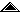Universidad de Costa Rica Escuela de Ciencias de la Computación e Informática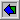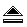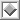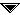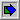# Yet Another C++ Money Class

## ResumenAn efficient and simple C++ class to handle `money` quantities is presented. The class uses scaled `double`'s to represent `money` data variables. Se presenta una eficiente y simple clase C++ para manipular datos que representan dinero. La clase se implementa usando números de punto flotante dobles.

I use C to program information systems where handling money quantities is quite common. Using `double`'s to represent money quantities is not good enough, because decimals are not represented correctly and sometimes round-off errors occur. A while ago a friend of mine gave me a very good idea to overcome this problem: he works using Canon BASIC, and he has always used `floor(double*100.0)` to represent money quantities. Any amount is represented as a `double` with no fractional part. With this trick no decimals are lost because the `double` type, with its `15+` digit precision, is used as a compiler supported `long long`. You need to be careful when multiplying and dividing, but in many applications these operations are rare.

For example, \$25.35 is represented as the `double` `2535.0`, using a scale factor of `100` (for two decimal places). The problem I kept facing in my C programs was to remember when to multiply by `100` and when not to. For example, to add two `double`'s that represent money quantities there is no need to multiply by `100`:

```\$25.35 + \$35.75 <==> 2535.0 + 3575.0 == 6110.0 <==> \$61.10
```

Also, one should never multiply by the scale factor when multiplying a `money` quantity:

```\$100 * (1.0+0.06) <==> 10000.0 * (1.06) == 10600.0 <==> \$106
```

It is just too easy to forget to multiply by the scale factor when using `double`'s as `money`. We human beings forget too much, so we need the computer to remember for us.

When I started programming in C++, I realized that a C++ `money` class was the solution for these problems. However, I needed my `money` class to be as efficient as possible; otherwise who would use it?

## Available toolsMy first move was to examine the available tools to handle money quantities. I looked at the following:
• Borland's C++ BCD class
• Zortech's C++ BCD class
• Zortech's C++ `money` class
BCD stands for Binary Coded Decimal. We frequently use binary representation, where a number is stored in a "computer word" as a sequence of binary "digits" that we call "bits". In BCD, what we store are the base 10 digits of a number, usually using their binary values. For example, the number `1234` will be stored in a pair of bytes using the same bit pattern as the `0x1234` hexadecimal constant. As most computers use eight bit bytes, each byte will hold two BCD digits. Many bit patterns are not valid when interpreted as BCD numbers: `0xFFFF`, `0x0A0A`, `0x123A`, are all invalid BCD quantities because in decimal notation we can use only the digits `0...9`.

I chose not to use Borland's BCD class for many reasons. It does not provide specific support to handle money quantities. For example, whenever you multiply and divide two `money` variables you have to figure out what happens with the remaining digits that go further than the number of cents. The header file in Borland C++ v2.0 sucks in the `<iostream.h>` header file which slows down compilation quite a bit. I still don't use streams, and I don't like to be forced to use them if I don't have to. As much of the BCD library needs to be loaded when using BCDs, this increases the size of executable files, which I find unattractive. Finally, the source code for this class is not included with the compiler (it is available at an extra charge); I have learned to examine the source code for a library before using it. The BCD class by Zortech shares all of these inconveniences.

In the Zortech C++ Tools package a `money` class is implemented, where a money quantity is a two member structure:

```class money {
long dollars;
int  cents;
// ...
};
```

In most implementations, a `long` can hold only nine or ten decimal digits, which means that the range of numbers that this class permits is smaller the range granted by `double`'s. Zortech's implementation is quite clean, but nonetheless bulky (or bulkier than mine, if you prefer). This class is efficient, because the operators are implemented using integer arithmetic and each `money` variable is quite short.

I know these tools are good enough to handle money quantities. Perhaps I didn't use them because I didn't write them. We programmers like to use our own tools, and when the cost of implementing them is not very big, we usually wind up rewriting code. I feel also that my experience using scaled `double`'s in C made me reluctant to use the available options, because those implementations were not meant to solve exactly my problem. I guess to be an inventor one needs to dislike what is already available...

Also, I wanted my `money` class to be portable, not compiler vendor dependent.

## Defining requirementsAfter examining these classes, I sat down to define the requirements for my `money` class. This is what I came up with:
1. Money quantities should behave as regular numbers.
2. The `money` class should be portable.
3. The programmer should be protected from misusing `money` quantities.
4. It should be possible to use standard library functions with `money` quantities.
5. Most operators should be inlined, to let the compiler optimize the generated code.
6. The `money` header file should be short.
7. The programmer should be able to define the number of decimals in a `money` data item.

These requirements are quite natural. Good object oriented programming dictates that a class represents a concept that we need to use in a straight-forward way; an efficient implementation is always welcomed by any C++ programmer. Where I had to give in was in the amount of storage that a `money` variable would use: eight bytes for most computers compared to six (25% less) if one uses the Zortech implementation.

Listing 1 is the header file `"money.h"` which defines and implements the `money` class. You will find amusing that all the methods in this class are `inline`: I did this to fulfill my fifth requirement. There are many versions of some of the arithmetic operators to cater for the various cases that are usually found in real life programs.

The `money` class permits a programmer to write expressions such as the following:

```money  mm,m = 1000;    // I've got a thou'
double tax  = 0.23;    // State Government of Insomnia
m *= (1-tax);          // This is what I have
m  = m+500;            // Thanks, mommy...
mm = 500;
m  = m + 1500 - (mm / m) * (1.0/3.0); // etc...
printf("Salary = %10.2f\n",
(double) ((10+mm)/m * m));
```
In a nutshell, the programmer can freely mix money quantities with regular numbers to get the correct results. Furthermore, the compiler will warn the programmer when he tries to misuse `money` data items, as in:
```money  m, mm;     // ok
double d = m*mm;  // can't multiply moneys
mm = d/m;         // can't divide by money
```
Listing 2 is a test program for the `money` class. You need to use your symbolic debugger to see what is going on at each point in the program.

## Implementation detailsI tried first to fake money quantities using `long`'s, but the difficulty in doing so stopped me from pursuing this approach (note that the Zortech tools `money` type is implemented using integer arithmetic).

As I knew that a `money` variable would be a `double`, the main problem to solve implementing this class was to keep track of when to multiply by the scale factor and when not to. This is accomplished by each of the overloaded arithmetic operators. I also implemented the `money::FIX()` member functions to get rid of the excess decimals whenever this is needed.

When the preprocesssor constant `MONEY_ROUNDING` is defined, the exccess decimals in a `double` are rounded when a `double` is assigned to a `money` variable. Otherwise the excess decimals are truncated:

```money  m(1.5199);  // \$1.52, when MONEY_ROUNDING
money  m(1.5199);  // \$1.51, when not MONEY_ROUNDING
```
The programmer cannot selectively choose whether to round or not case by case: it is an all or nothing affair because the decision must be made at compile time.

The preprocessor constant `MONEY_DECIMALS` defines how many decimals a `money` item has. The member function `money::SCALE()` is implemented using a preprocessor trick that returns the scale factor used to multiply a `double` to make it a `money` quantity. If three decimals are needed for `money` items, then the scale factor would be `1,000 = 10^3`. In some countries the inflation is so high that the number of decimals is negative; in this case the scale factor would be a number less than one. As `money::SCALE()` is an `inline` function, the compiler can optimize out the division by the scale factor in some cases. If the programmer doesn't define `MONEY_DECIMALS`, then a default value of 2 decimals is used. In my programs, I define `MONEY_DECIMALS` before including the `<money.h>` file:

```#define   MONEY_DECIMALS 4  // must use a decimal number
#include "money.h"          // or TENPOW bombs.
```
The vector constructor `money::money()` does not initialize a `money` item, because in many cases doing so is wasteful. Money items can be regarded by the programmer as regular numbers. The compiler should be able to optimize out this constructor if it is used.

The class `money` implements most arithmethic operators, but it does not implement the following:

```money operator* (const money&, const money&);
money operator/ (const double, const money&);
```
It just does not make sense to use them in a program; if you use them you will get a compile time error (a disatisfied programmer could add them to the class easily).

A careful examination of the implementation of each of the arithmetic operators in file `"money.h"` will show that the class is programmed to minimze the number of times that each `double` needs to be scaled up by the scale factor.

The comparison operators are defined only for `money` items. This means that when a `money` data type is compared to a `double`, the compiler will promote the `double` to a `money` variable using the constructor `money::money(double)`. If this is not what the programmer intended, an explicit typecast can be used:

```double d = 15.253;     //   15.253
money  m = 15.25;      // \$ 15.25

if (d == m) {          // TRUE:  d becomes money(d)
}
if (d == (double) m) { // FALSE: 15.253 != 15.25
}
```
The function `flatten(money, cents, rounding)` is very useful to round up a money quantity to the nearest value that can be paid with coins. For example, in Costa Rica there are no one cent coins, because the smallest coin is worth 25 cents of a colón (which we call a "peseta"). In the following example a `money` item is rounded up to pesetas:
```money m  = 125.80;      // ¢ 125.80 colones
money mm = flatten(m);  // ¢ 125.75 colones
```
The complete `money` class is implemented in the header file called `"money.h"` using `inline` functions, to permit the compiler to optimize out any floating point operations when it can. I decided not to provide more operators for `money` items, because the type converter `money::operator double()` allows the standard math functions to be used with `money` items. The header file `<money.h>` includes only two files, `<math.h>` and `<float.h>`, which have the prototypes for functions `floor()`, `ceil()`, `fmod()`, and `DBL_DIG`. If you want to, you can declare every `double` variable in `"money.h"` as a `long double`, to use bigger `money` quantities.

As implemented, the `money` class should be quite portable because it does not make use of any odd C++ constructs. Depending on the compiler being used, it is quite possible to optimize out many of the `inline` operators, and thus yield efficient programs.

## ConclusionThe `money` class is a tiny C++ class that lets the programmer use `money` items with ease. The implementation is as efficient as using floating point values in arithmetic expressions. There is no reason to prevent you from using it right away.

Small is beautiful.

## FundingEsta investigación se realizó dentro del proyecto de investigación 326-89-019 "Estudios de la Tecnología de Programación por Objetos y C++", inscrito ante la Vicerrectoría de Investigación de la Universidad de Costa Rica. La Escuela de Ciencias de la Computación e Informática también ha aportado fondos para realizar este trabajo.

## AcknowledgmentsCarlos Loría gave me the initial idea to develop this class. He also took the time to review the preliminary versions of this paper.

## Listing 1: The `money` ClassTo obtain an ASCII version of the following files you have the following options:
• Save the whole file from the browser as an ASCII file, and edit out all the text for the article, using the text editor of your preference.
• Mark the section of code you want, to cut & paste it elsewere.
• Get these files directly from directory ``` http://www.di-mare.com/adolfo/p/src ```
``` http://www.di-mare.com/adolfo/p/src/money.h```
``` http://www.di-mare.com/adolfo/p/src/money.c```
```/* @(#) money.h          Copymiddle 1991 Adolfo Di Mare  */
/*                                                       */
/*            Yet Another Money C++ Class                */
/*                                                       */
/*   Use freely but acknowledge author and publication.  */
/*    DO NOT SELL IT. The author reserves all rigths!    */

/* Compiler:                           Borland C++ v 2.0 */
/*                          [should work with Turbo C++] */

#ifndef _money_h
#define _money_h

extern "C" {     // avoid type clashes with the C library
#include <math.h>    /* floor() */
#include <float.h>   /* DBL_DIG */
}

#ifndef MONEY_DECIMALS       /* number of decimals in */
#define MONEY_DECIMALS  2    /*   any money quantity  */
#endif                       /* don't use parentesis! */

#define __VAL(n)  n               /* 1 level indirection */
#define TENPOW(n) __VAL(1.0e##n)  /* Trick to yield 10^n */

#define MONEY_DIG   DBL_DIG

class money {
public:

static int    decimals() { return MONEY_DECIMALS;     }
static int    digits()   { return MONEY_DIG; }
static double SCALE()
{ return TENPOW(MONEY_DECIMALS); }

money();              // do nothing constructor
money(double);        // constructor from double
money(const money&);  // copy constructor

money& operator= (const money&);  // copy operator
money& operator= (double);        // copy from double
operator double() const;          // convert to double

int  OK()   const;  // check money's invariant
void FIX();         // get rid of unwanted decimals

friend money  operator + (const money&, const money&);
friend money  operator + (double,       const money&);
friend money  operator + (const money&, double);
friend money  operator - (const money&, const money&);
friend money  operator - (double,       const money&);
friend money  operator - (const money&, double);

friend money  operator*  (const money&, double);
friend money  operator*  (double,       const money&);
friend double operator/  (const money&, const money&);
friend money  operator/  (const money&, double);
friend money  operator%  (const money&, const money&);

// money  * money  is NOT valid
// double / money  is INVALID

friend int operator == (const money&, const money&);
friend int operator != (const money&, const money&);
friend int operator <  (const money&, const money&);
friend int operator >  (const money&, const money&);
friend int operator <= (const money&, const money&);
friend int operator >= (const money&, const money&);

friend int operator == (const money&, double);
friend int operator != (const money&, double);
friend int operator <  (const money&, double);
friend int operator >  (const money&, double);
friend int operator <= (const money&, double);
friend int operator >= (const money&, double);

friend int operator == (double, const money&);
friend int operator != (double, const money&);
friend int operator <  (double, const money&);
friend int operator >  (double, const money&);
friend int operator <= (double, const money&);
friend int operator >= (double, const money&);

money& operator += (const money&);
money& operator += (double);
money& operator -= (const money&);
money& operator -= (double);

money& operator *= (double);
money& operator /= (double);

friend money  operator+ (const money&);
friend money  operator- (const money&);
money& operator++();     // prefix
money& operator--();
money& operator++(int);  // postfix
money& operator--(int);
friend int    operator! (const money&);

friend money abs(const money&);
friend money flatten(
const money& m,
double cents=0.25, int rounding = 1 /* TRUE */);

protected:         // let users change the class behaviour
double m_money;
};

// Constructors && assignment
inline money::money() {
// do nothing constructor, for efficiency
}
inline money::money(double d) {
// construct from double
m_money = d*SCALE();
FIX();
}
inline money::money(const money& m) {
// copy constructor
m_money = m.m_money;
}

inline money& money::operator= (const money& m) {
// copy operator
m_money = m.m_money;
return *this;
}
inline money& money::operator= (double d) {
// assign from double
m_money = d*SCALE();
FIX();
return *this;
}
inline money::operator double() const {
// convert to double
return m_money / SCALE();
}

inline int money::OK() const {
// Returns TRUE (1) when the quantity stored
// in *this really corresponds to a money
// quantity.

money temp;
temp.m_money = m_money;
temp.FIX();
return (
( temp.m_money == m_money )
&&
( fabs(m_money) < (TENPOW(DBL_DIG) / SCALE()) )
);
}

inline void money::FIX() {
// Deletes all decimals digits beyond
// the MONEY_DECIMALS decimal place.
// - If the value is out of range, FIX
//   won't fix it.
m_money =
(m_money > 0.0
?
floor(
m_money
#ifdef MONEY_ROUNDING
+ 0.5 // 0.49 is also an option...
#endif
)
:
ceil(
m_money
#ifdef MONEY_ROUNDING
- 0.5
#endif
)
);
}

inline money operator+ (const money& m, const money& mm) {
money temp;    // don't mult*SCALE()
temp.m_money = m.m_money + mm.m_money;
return temp;
}
inline money operator+ (double d, const money& m) {
return (money(d)+m);
}
inline money operator+ (const money& m, double d) {
return (m+money(d));
}

// substract
inline money operator- (const money& m, const money& mm) {
money temp;
temp.m_money = m.m_money - mm.m_money;
return temp;
}
inline money operator- (double d, const money& m) {
return (money(d)-m);
}
inline money operator- (const money& m, double d) {
return (m-money(d));
}

// multiply
inline money operator* (const money& m, double d) {
money temp;
temp.m_money = m.m_money * d; // don't mult by SCALE()
temp.FIX();    // this could be delayed...
return temp;
}
inline money operator* (double d, const money& m) {
return (m*d);
}

// divide
inline double operator/ (const money& m, const money& mm) {
return m.m_money / mm.m_money;
}
inline money  operator/ (const money& m, double d) {
money temp;
temp.m_money = m.m_money / d;
temp.FIX();    // this could be delayed...
return temp;
}
inline money operator%  (const money& m, const money& mm) {
money temp;
temp.m_money = fmod(m.m_money, mm.m_money);
temp.FIX();    // this could be delayed...
return temp;
}

// compare
inline int operator  == (const money& m, const money& mm) {
return m.m_money ==  mm.m_money;
}
inline int operator  != (const money& m, const money& mm) {
return m.m_money !=  mm.m_money;
}
inline int operator  <  (const money& m, const money& mm) {
return m.m_money <   mm.m_money;
}
inline int operator  >  (const money& m, const money& mm) {
return m.m_money >   mm.m_money;
}
inline int operator  <= (const money& m, const money& mm) {
return m.m_money <=  mm.m_money;
}
inline int operator  >= (const money& m, const money& mm) {
return m.m_money >=  mm.m_money;
}

inline int operator  == (const money& m, double mm) {
return m.m_money == mm;
//  return m.m_money == (money)mm;  // take a pick !!!
/*
A decission that you should make is whether this
equality comparison requires the double quantity
to  be  promoted  to  a money item.  The  direct
comparison   is   more   transparent,   so it is
prefered in here.
*/
}
inline int operator  != (const money& m, double mm) {
return !(m == mm);
}
inline int operator  <  (const money& m, double mm) {
return m.m_money <  mm;
}
inline int operator  >  (const money& m, double mm) {
return m.m_money >  mm;
}
inline int operator  <= (const money& m, double mm) {
return m.m_money <= mm;
}
inline int operator  >= (const money& m, double mm) {
return m.m_money >= mm;
}

inline int operator  == (double m, const money& mm) {
return  (mm == m);
}
inline int operator  != (double m, const money& mm) {
return !(mm == m);
}
inline int operator  <  (double m, const money& mm) {
return         m <  mm.m_money;
}
inline int operator  >  (double m, const money& mm) {
return         m >  mm.m_money;
}
inline int operator  <= (double m, const money& mm) {
return         m <= mm.m_money;
}
inline int operator  >= (double m, const money& mm) {
return         m >= mm.m_money;
}

inline money& money::operator += (const money& m) {
m_money += m.m_money;
return *this;
}
inline money& money::operator += (double d) {
m_money += d*SCALE();
FIX();
return *this;
}
inline money& money::operator -= (const money& m) {
m_money -= m.m_money;
return *this;
}
inline money& money::operator -= (double d) {
m_money -= d*SCALE();
FIX();
return *this;
}
inline money& money::operator *= (double d) {
m_money *= d;
FIX();
return *this;
}
inline money& money::operator /= (double d) {
m_money /= d;
FIX();
return *this;
}

// unary op's
inline money operator+(const money& m) {
return m;
}
inline money operator-(const money& m) {
money temp;
temp.m_money = -m.m_money;
return temp;
}
inline money& money::operator++() {
m_money += SCALE();
#if (MONEY_DECIMALS<0)
FIX(); // avoid problems because of
#endif     // the representation of 10^-n
return *this;
}
inline money& money::operator--() {
m_money -= SCALE();
#if (MONEY_DECIMALS<0)
FIX();
#endif
return *this;
}

inline money& money::operator++(int) {
return ++(*this);
}

inline money& money::operator--(int) {
return --(*this);
}
inline int operator!(const money& m) {
return m.m_money == 0.0;
}

inline money abs(const money& m) {
money temp;
temp.m_money = fabs(m.m_money);
return temp;
}

money flatten(const money& m, double cents, int rounding) {
// Returns a money data item where the cents are
// rounded modulo "cents". In this way cents can
// be stripped of money items when the currency
// does not have all the coins required to pay
// every posible quantity.
money temp;
double c = floor(fabs(cents*money::SCALE())); // cents
double r = fmod(m.m_money, c);            // remainder
temp.m_money =
(!rounding || (2.0* r <= c)
? m.m_money - r
: m.m_money - r + c
);
return temp;
}

/* Avoid name space overcrowding */
#undef __VAL
#undef TENPOW /* jic: Just In Case! */

#endif  /* _money_h */
```

## Listing 2: Test driver for "`money.h`"```/* @(#) money.c                     1991 Adolfo Di Mare  */
/*                                                       */
/*                Test driver for money.h                */
/*                                                       */
/* Compiler:                           Borland C++ v 2.0 */
/*                          [should work with Turbo C++] */

/*
To see what is going on, you need to use your symbolic
debugger to examine each of the declared variables.

For Borland C++, I used the following watches:

m_money      d,f18
m,r          i
mm,r         l
elapsed      s,f18

Change the compile time macros to see how money's
change their behaviour.
*/

#define TEST

#ifdef  TEST /* { */

#if 0
#define MONEY_ROUNDING    /* Force rounding of doubles */
#endif

#define MONEY_DECIMALS  2   /* 2 decimals for money data */

extern "C++" {
#include "money.h"

#ifdef __TURBOC__
#include <iostream.h>
#else
#include <stream.h>
#endif
}

inline ostream& operator<<(ostream &out, money& m) {
return out << (double) m;
}

extern "C" {
#include <stdlib.h>
#include <time.h>
#include <stdio.h>
}

#define RANGER
#undef  RANGER           /* takes forever to run...   */

void ranger(void);

void main(void) {

// To the right is the assigned value

money m(25.8499); // \$  25.84
money mm;         // \$ #\$\$#@\$@.98

int     i = 5;    //     5
long    l = 6;    //     7
double  d = m;    //    25.8399999999999999999999999999

i  = m;           //    25
#if 0     /* [ */
m  = d;           // \$  25.84
m  = 7.009999999; // \$   7.oo
m += 1;           // \$   8.oo
m += 1.245000001; // \$   9.24
m += 1L;          // \$  10.24
m += 'a';         // \$  'a'+10.24

m  = -d;          // \$ -25.84
m  = -7.00999999; // \$ - 7.oo
m -= 1;           // \$ - 8.oo
m -= 1.245000001; // \$ - 9.24
m -= 1L;          // \$ -10.24
m -= 'a';         // \$ -'a'-10.24

mm = 10;          // \$  10.oo
m  = mm+4;        // \$  14.oo
m  = mm+4.014999; // \$  14.01
m  = 4.99+mm;     // \$  14.99
m  = 4+mm;        // \$  14.oo
m  = m+mm;        // \$  24.oo
m += m;           // \$  48.oo

d  = mm+4;        //    14.oo
d  = mm+4.011;    //    14.0099999999999998
d  = 4.99+mm;     //    14.9900000000000002
d  = 4+mm;        //    14.oo

mm = 10;          // \$  10.oo
m  = mm*4;        // \$  40.oo
m  = mm*4.0;      // \$  40.oo
m  = 4*mm;        // \$  40.oo
m  = 4.0*mm;      // \$  40.oo

mm = 10;          // \$  10.oo
d  =  7.00001;    //     7.00000999999999962
m  = d*mm+d;      // \$  77.oo
m  = (d*mm)+d;    // \$  77.oo
m  = d+d*mm;      // \$  77.oo
m  = d+(d*mm);    // \$  77.oo

m = 10;           // \$  10.oo
mm = 77;  // \$  77.oo
m = m % mm;       // \$  10.oo
// \$10 == 0L * \$77 + [\$10]

m = 77;           // \$  77.oo
mm = 10; // \$  10.oo
m = m % mm;       // \$   7.oo
// \$77 == 7L * \$10 + [\$7]

m++;              // \$   8.oo
m--;              // \$   7.oo

m   = 11.75;                // \$  11.75
m  +=  0.12;                // \$  11.87
mm  = flatten(m,0.25,1);    // \$  11.75

m  += 0.01;                 // \$  11.88
mm  = flatten(m,0.25,1);    // \$  12.oo

m   = 11.75;                // \$  11.75
m  +=  0.12;                // \$  11.87
mm  = flatten(m,0.25,0);    // \$  11.75

m  += 0.01;                 // \$  11.88
mm  = flatten(m,0.25,0);    // \$  11.75

m  -= 5;
m  += 0.12;                 // \$   7.oo
if (m == 0 || 0 == m) {     // nep
m += d;
}
else if (!(m == m)) {       // nep
m = m;
}
else if (m > m) {           // nep
m = m;
}
else if (m < m) {           // nep
m = m;
}
else if (m != m) {          // nep
m = m;
}
else if (m >= m) {          // yep
m *= 11;      // \$  77.oo
m += 15;      // \$  92.oo
}
l  = i = m;       //    92
m  = -m;          // \$ -92.oo
mm = i*l;         // \$   8,464.oo
m  = m % mm;      // \$ -92.oo

d  = 15.253;      //   15.253
m  = 15.25;       // \$ 15.25

if (d == m) {          // FALSE: float comparison...
l = 0;
}                      // l = 92L
if (d == (double) m) { // FALSE: 15.253 != 15.25
l++;
}                      // l = 92L

// simulate a TAX calculation
m = 0.0;
for (i = 1; i <= 100; i++) {
d  = i*1.005;    // 0.05% tax
m += d;
}                    // \$ 5,075.oo
mm = 100;            // \$   100.oo
m /= (double) mm;    // \$    50.75
m /=   3;            // \$    16.91
m *=   3;            // \$    50.73

mm = mm/mm;          //       1.00
d  = m * (m / mm);   //    2573.5300000000002

d  = 1.0/3.0 * m;    //      16.9100000000000001
mm = 1.0/3.0 * m;    // \$    16.91

mm = 3 * mm / (3 * mm);                  // \$1.oo
mm = M_PI * mm / (M_PI * mm);            // \$1.oo
mm = M_PI;                               // \$3.14

mm = mm/mm + 1 - (3 * mm / (3 * mm));    // \$1.oo
m *= M_E * mm / (mm * M_E) - 1;          // \$0.oo

// m == 0.0 && mm == \$1.oo
for (i = 1; i <= 100; i++) {
mm /= 3;          // \$    0.33
m   = m+mm;       // Add a third
mm  = 1;
}                     // \$    33.oo

d  = m;               //      33.00
mm = m / 330;         // \$     0.10

clock_t now;
double  elapsed;

// time statistics, on a 33 MHz 386
m = 0;
now = clock();
for (l = 0; l <= 10000l; l++) {
m += 1.01;          // Add \$1.01
}
elapsed = (clock()-now) / CLK_TCK;
d  = elapsed;           // 3.51 secs

m = 1;
now = clock();
for (l = 0; l <= 10000l; l++) {
m *= 1.0001;        // Mult
}
elapsed = (clock()-now) / CLK_TCK;
d  = elapsed;           // 3.24 secs

m = 1;
mm = pow(10, 6);
now = clock();
for (l = 0; l <= 10000l; l++) {
mm = m;
m /= 0.99001;       // Div
if (!m.OK()) {
m.FIX();        // won't fix overflows
}
}
elapsed = (clock()-now) / CLK_TCK;
d  = elapsed;           //  8.46 secs

d  = m % m + 33;        //   33.00
m  = d;                 // \$ 33.oo
mm = d / 330;           // \$  0.10

d = (10+mm)/m * m;      //   10.089...

// Must use (double) type cast
printf ("Salary = %10.2f\n",
(double) ((10+mm)/m * m));
cout <<"Salary = "
<< (double) ((10+mm)/m * m) << '\n';

// valid only if you define
// ostream& operator<< (ostream&, money&)
cout <<"Salary = "
<< (10+mm)/m * m << '\n';

//  m = d / m;        // should not compile...
//  m = m * m;        // won't compile: AMBIGUITY ERROR!!!

#ifdef  RANGER
ranger();
#endif // RANGER

#endif    /* ] */
exit(0);
}

void ranger(void) {
/*
Shows that indeed a double can hold up to
DBL_DIG digits of precission.
*/
// This should take forever to calculate...
char view[] = "0123456789/123456";
double s,t;
double tenpow,inc;
int    i;

tenpow = pow(10, DBL_DIG); // 10^15
inc    = 100.0;            // pick yours

s = floor(tenpow+inc); // 1,000,000,000,000,000 + inc
for (;;) {
t = s;
s += inc;
//      i = (int) log10(t-tenpow);
if (s == t) {
i = (int) log10(t-tenpow);
cout << "BOOM t   = " << t      << '\n';
cout << "BOOM s   = " << s      << '\n';
cout << "BOOM inc = " << s      << '\n';
cout << "BOOM i   = " << i      << '\n';
view[i] = 0;
cout << "view     = " << view   << '\n';
return;
}
}

return;
} // ranger

#endif  /* TEST */  /* } */
```

## BibliographyWright, Maynard A.: Complex Function Library, The C Users Journal, Vol.8 No.9, pp [45-53], September 1990.  Shapiro, Jim: Whe have mail, The C Users Journal, (a comment on ), Vol.9 No.5, pp [142,144], May 1991.  Timothy Prince: Pennies in Long Double, The C Users Journal, Vol.9 No.1, pp [77-78,83-84,86], January 1991.  Smith III, Thad: Whe have mail, The C Users Journal, (a comment on ), Vol.9 No.4, pp [126,128], April 1991.

## Index[-] Abstract [-] Available tools [-] Defining requirements [-] Implementation details [-] Conclusion [-] Funding [-] Acknowledgments [-] Listing 1: The `money` Class [-] Listing 2: Test driver for "`money.h`"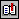BibliographyIndice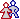Acerca del autor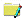Acerca de este documentoPrincipioIndiceFinal

## Acerca del autorAdolfo Di Mare: Investigador costarricense en la Escuela de Ciencias de la Computación e Informática [ECCI] de la Universidad de Costa Rica [UCR], en donde ostenta el rango de Profesor Catedrático. Trabaja en las tecnologías de Programación e Internet. Es Maestro Tutor del Stvdivm Generale de la Universidad Autónoma de Centro América [UACA], en donde ostenta el rango de Catedrático y funge como Consiliario Académico. Obtuvo la Licenciatura en la Universidad de Costa Rica y la Maestría en Ciencias en la Universidad de California, Los Angeles [UCLA].

## Acerca de este documentoReferencia: Di Mare, Adolfo: Yet Another C++ Money Class, The C Users Journal, Vol.10 No.4, pp [58-64], April 1992. Internet: ``` http://www.di-mare.com/adolfo/p/money.htm ``` Autor: Adolfo Di Mare Contacto: Apdo 4249-1000, San José Costa Rica Tel: (506) 207-4020       Fax: (506) 438-0139 Revisión: ECCI-UCR, Octubre 1997 Visitantes: